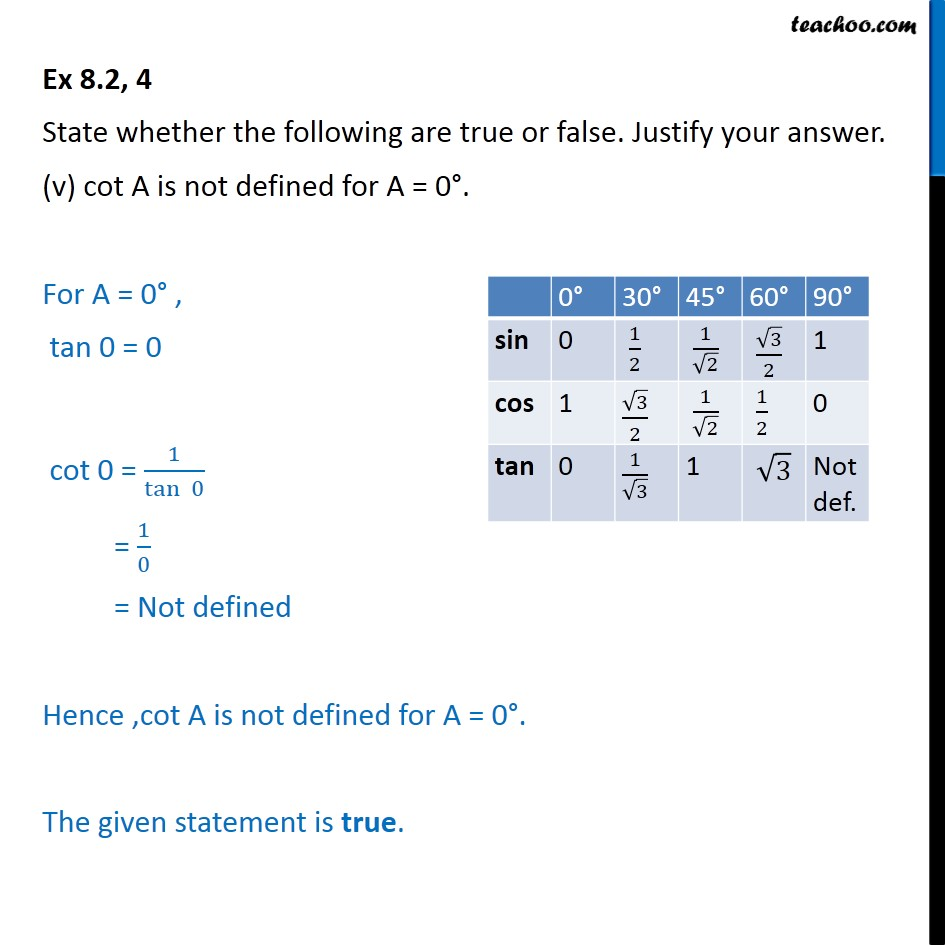1. Chapter 8 Class 10 Introduction to Trignometry (Term 1)
2. Serial order wise
3. Ex 8.2

Transcript

Ex 8.2, 4 State whether the following are true or false. Justify your answer. (v) cot A is not defined for A = 0°. For A = 0° , tan 0 = 0 cot 0 = 1/(tan⁡ 0) = 1/0 = Not defined Hence ,cot A is not defined for A = 0°. The given statement is true.